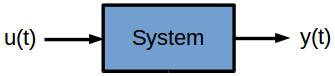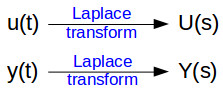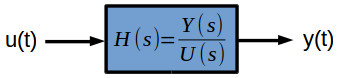# How to find the transfer function of a system

In control engineering and control theory the transfer function of a system is a very common concept. A transfer function is determined using Laplace transform and plays a vital role in the development of the automatic control systems theory.

By the end of this tutorial, the reader should know:

• how to find the transfer function of a SISO system starting from the ordinary differential equation
• how to simulate a transfer function in an Xcos block diagram
• how to simulated a transfer function using Scilab dedicated functions

A system can be defined as a mathematical relationship between the input, output and the states of a system. In control theory, a system is represented a a rectangle with an input and output.where:

u(t) – input
y(t) – output

Both input and output are variable in time. A system with only one input and output is called SISO (Single Input Single Output) system. Control theory also applies to MIMO (Multi Input Multi Output) systems, but for an easier understanding of the concept we are going to refer only to SISO systems.

The simplest representation of a system is through Ordinary Differential Equation (ODE). When dealing with ordinary differential equations, the dependent variables are function of a positive real variable t (often time). By applying Laplace’s transform we switch from a function of time to a function of a complex variable s (frequency) and the differential equation becomes an algebraic equation.The transfer function defines the relation between the output and the input of a dynamic system, written in complex form (s variable). For a dynamic system with an input u(t) and an output y(t), the transfer function H(s) is the ratio between the complex representation (s variable) of the output Y(s) and input U(s).For a better understanding we are going to have a look at two example, two dynamic systems, for which we are going to find (determine) their transfer functions. These systems are:

### Laplace Transform of Derivative

Before going into practical examples, let’s recall Laplace transform for a function, first order derivative and second order derivative.

For a given continuous and differentiable function f(t), the following Laplace transforms properties applies:

$\begin{split} \mathcal {L} [f(t)] &= F(s)\\ \mathcal {L} [f'(t)] &= sF(s)-f(0)\\ \mathcal {L} [f”(t)] &= s^2F(s)-sf(0)-f'(0) \end{split}$

### Mechanical system

Example. Find the transfer function for a single translational mass system with spring and damper.

The methodology for finding the equation of motion for this is system is described in detail in the tutorial Mechanical systems modeling using Newton’s and D’Alembert equations.

The ordinary differential equation describing the dynamics of the system is:

$F(t) = m \frac{d^2 x(t)}{dt^2}+c \frac{dx(t)}{dt}+ kx(t) \tag{1}$

where:

m [kg] – mass
k [N/m] – spring constant (stiffness)
c [Ns/m] – damping coefficient
F [N] – external force acting on the body (input)
x [m] – displacement of the body (output)

The input of the system is the external force F(t) and the output is the displacement x(t). First we’ll apply the Laplace transform to each of the terms of the equation (1):

$\begin{split} \mathcal {L} \left [ \frac{d^2 x(t)}{dt^2} \right ] &= s^2 X(s)-sx(0)- \frac{dx(0)}{dt}\\ \mathcal {L} \left [ \frac{d x(t)}{dt} \right ] &= s X(s)-x(0)\\ \mathcal {L} \left [ x(t) \right ] &= X(s)\\ \mathcal {L} [F(t)] &= F(s)\\ \end{split}$

The initial conditions of the mass position and speed are:

$\begin{split} x(0) &= 0\\ \frac{dx(0)}{dt} &= 0 \end{split}$

Replacing the Laplace transforms and initial conditions in the equation (1) gives:

$\begin{split} F(s) &= ms^2X(s)+csX(s)+kX(s)\\ F(s) &= X(s) \left ( ms^2 + cs + k \right )\\ \frac{X(s)}{F(s)} &= \frac{1}{ms^2 + cs + k} \end{split}$

We have now found the transfer function of the translational mass system with spring and damper:

$\bbox[#FFFF9D]{H(s) =\frac{X(s)}{F(s)} =\frac{1}{ms^2 + cs + k}}$

To prove that the transfer function was correctly calculated, we are going to use a simple Xcos block diagram to simulate the step response of the system.

With the following parameters:

m = 2; // [kg]
c = 1; // [Ns/m]
k = 2; // [N/m]

and running the Xcos simulation for 20 s, gives the following graphical window:

The response given by the transfer function is identical with the response obtained by integrating the ordinary differential equation of the system. This gives confidence in the calculation method for the transfer function.

We could also use the Scilab function syslin() to define a transfer function. Also, with the function csim(), we can plot the system’s response to a unitary step input.

// Define system parameters
m = 2; // [kg]
c = 1; // [Ns/m]
k = 2; // [N/m]

// Define simulation time
t=0:0.01:20; // [s]

// Define transfer function
s=poly(0,'s');
H=[1/(m*s^2+c*s+k)];
sys=syslin('c',H)

// Run unitary step response
x_m = csim('step',t,H);
plot(t,x_m), xgrid()
xlabel('t [s]')
ylabel('x [m]')

By running the above Scilab instructions, we get the following graphical window:

As expected, we have the same system response as in the Xcos block diagram transfer function simulation.

We have now defined the same mechanical system as a differential equation and as a transfer function. Both representations are correct and equivalent.

### Electrical system

Example. Find the transfer function of a series RL circuit connected to a continuous current voltage source.

The methodology for finding the electrical current equation for the system is described in detail in the tutorial RL circuit – detailed mathematical analysis.

The ordinary differential equation describing the dynamics of the RL circuit is:

$u(t) = L \frac{di(t)}{dt} +R i(t) \tag{2}$

where:

R [Ω] – resistance
L [H] – inductance
u [V] – voltage drop across the circuit
i [A] – electrical current through the circuit

The input of the system is the voltage u(t) and the output is the electrical current i(t). First we’ll apply the Laplace transform to each of the terms of the equation (2):

$\begin{split} \mathcal {L} \left [ \frac{d i(t)}{dt} \right ] &= s I(s)-i(0)\\ \mathcal {L} \left [ i(t) \right ] &= I(s)\\ \mathcal {L} [u(t)] &= U(s)\\ \end{split}$

The initial condition of the electrical current is:

$i(0) = 0$

Replacing the Laplace transforms and initial conditions in the equation (2) gives:

$\begin{split} U(s) &= LsI(s)+RI(s)\\ U(s) &= I(s) \left ( Ls + R \right )\\ \frac{I(s)}{U(s)} &= \frac{1}{Ls + R} \end{split}$

We have now found the transfer function of the series RL circuit:

$\bbox[#FFFF9D]{H(s) =\frac{I(s)}{U(s)} =\frac{1}{Ls + R}}$

To prove that the transfer function was correctly calculated, we are going to use a simple Xcos block diagram to simulate the step response of the system.

With the following parameters:

E = 12; // [V]
R = 0.3; // [Ohm]
L = 0.04; // [H]

and running the Xcos simulation for 2 s, gives the following graphical window:

The response given by the transfer function is identical with the response obtained by integrating the ordinary differential equation of the system. This gives confidence in the calculation method for the transfer function.

We could also use the Scilab function syslin() to define a transfer function. Also, with the function csim(), we can plot the system’s response to voltage step input.

// Define system parameters
E = 12; // [V]
R = 0.3; // [Ohm]
L = 0.04; // [H]

// Define simulation time
t = 0:0.01:2; // [s]

// Define transfer function
s=poly(0,'s');
H=[1/(L*s+R)];
sys=syslin('c',H)

// Define input function
deff('u=step(t)','u=E')

// Run step response
x_m = csim(step,t,H);
plot(t,x_m), xgrid()
xlabel('t [s]')
ylabel('i [A]')

By running the above Scilab instructions, we get the following graphical window:

As expected, we have the same system response as in the Xcos block diagram transfer function simulation.

We have now defined the same electrical system as a differential equation and as a transfer function. Both representations are correct and equivalent.

At the end of this tutorial, the reader should know:

• how to find the transfer function of a SISO system starting from the ordinary differential equation
• how to simulate a transfer function in an Xcos block diagram
• how to simulated a transfer function using Scilab dedicated functions

For any questions, observations and queries regarding this article, use the comment form below.

Don’t forget to Like, Share and Subscribe!

#### Ad Blocker Detected

Dear user, Our website provides free and high quality content by displaying ads to our visitors. Please support us by disabling your Ad blocker for our site. Thank you!